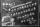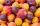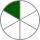# Fraction calculator

The calculator performs basic and advanced operations with fractions, expressions with fractions combined with integers, decimals, and mixed numbers. It also shows detailed step-by-step information about the fraction calculation procedure. Solve problems with two, three, or more fractions and numbers in one expression.

## Result:

### 11/12 + 5/3 + 1/4 = 17/6 = 2 5/6 ≅ 2.8333333

Spelled result in words is seventeen sixths (or two and five sixths).

### How do you solve fractions step by step?

1. Add: 11/12 + 5/3 = 11/12 + 5 · 4/3 · 4 = 11/12 + 20/12 = 11 + 20/12 = 31/12
For adding, subtracting, and comparing fractions, it is suitable to adjust both fractions to a common (equal, identical) denominator. The common denominator you can calculate as the least common multiple of both denominators - LCM(12, 3) = 12. In practice, it is enough to find the common denominator (not necessarily the lowest) by multiplying the denominators: 12 × 3 = 36. In the following intermediate step, the fraction result cannot be further simplified by canceling.
In other words - eleven twelfths plus five thirds = thirty-one twelfths.
2. Add: the result of step No. 1 + 1/4 = 31/12 + 1/4 = 31/12 + 1 · 3/4 · 3 = 31/12 + 3/12 = 31 + 3/12 = 34/12 = 2 · 17/2 · 6 = 17/6
For adding, subtracting, and comparing fractions, it is suitable to adjust both fractions to a common (equal, identical) denominator. The common denominator you can calculate as the least common multiple of both denominators - LCM(12, 4) = 12. In practice, it is enough to find the common denominator (not necessarily the lowest) by multiplying the denominators: 12 × 4 = 48. In the following intermediate step, cancel by a common factor of 2 gives 17/6.
In other words - thirty-one twelfths plus one quarter = seventeen sixths.

#### Rules for expressions with fractions:

Fractions - use the slash “/” between the numerator and denominator, i.e., for five-hundredths, enter 5/100. If you are using mixed numbers, be sure to leave a single space between the whole and fraction part.
The slash separates the numerator (number above a fraction line) and denominator (number below).

Mixed numerals (mixed fractions or mixed numbers) write as non-zero integer separated by one space and fraction i.e., 1 2/3 (having the same sign). An example of a negative mixed fraction: -5 1/2.
Because slash is both signs for fraction line and division, we recommended use colon (:) as the operator of division fractions i.e., 1/2 : 3.

Decimals (decimal numbers) enter with a decimal point . and they are automatically converted to fractions - i.e. 1.45.

The colon : and slash / is the symbol of division. Can be used to divide mixed numbers 1 2/3 : 4 3/8 or can be used for write complex fractions i.e. 1/2 : 1/3.
An asterisk * or × is the symbol for multiplication.
Plus + is addition, minus sign - is subtraction and ()[] is mathematical parentheses.
The exponentiation/power symbol is ^ - for example: (7/8-4/5)^2 = (7/8-4/5)2

#### Examples:

subtracting fractions: 2/3 - 1/2
multiplying fractions: 7/8 * 3/9
dividing Fractions: 1/2 : 3/4
exponentiation of fraction: 3/5^3
fractional exponents: 16 ^ 1/2
adding fractions and mixed numbers: 8/5 + 6 2/7
dividing integer and fraction: 5 ÷ 1/2
complex fractions: 5/8 : 2 2/3
decimal to fraction: 0.625
Fraction to Decimal: 1/4
Fraction to Percent: 1/8 %
comparing fractions: 1/4 2/3
multiplying a fraction by a whole number: 6 * 3/4
square root of a fraction: sqrt(1/16)
reducing or simplifying the fraction (simplification) - dividing the numerator and denominator of a fraction by the same non-zero number - equivalent fraction: 4/22
expression with brackets: 1/3 * (1/2 - 3 3/8)
compound fraction: 3/4 of 5/7
fractions multiple: 2/3 of 3/5
divide to find the quotient: 3/5 ÷ 2/3

The calculator follows well-known rules for order of operations. The most common mnemonics for remembering this order of operations are:
PEMDAS - Parentheses, Exponents, Multiplication, Division, Addition, Subtraction.
BEDMAS - Brackets, Exponents, Division, Multiplication, Addition, Subtraction
BODMAS - Brackets, Of or Order, Division, Multiplication, Addition, Subtraction.
GEMDAS - Grouping Symbols - brackets (){}, Exponents, Multiplication, Division, Addition, Subtraction.
Be careful, always do multiplication and division before addition and subtraction. Some operators (+ and -) and (* and /) has the same priority and then must evaluate from left to right.

## Fractions in word problems:

• In fractionsAn ant climbs 2/5 of the pole on the first hour and climbs 1/4 of the pole on the next hour. What part of the pole does the ant climb in two hours?
• Scouts 44/7 of the students in a school are boys. If 3/8 of the boys are scouts, how many scouts are there in a school of 1878 students?
• Unknown numberI think the number - its sixth is 3 smaller than its third.
• InqualitySolve inequality: 3x + 6 > 14
• Unknown number 6Determine x if 1/6 of x is equal to 2/5 of the number 24.
• DividendsThe three friends divided the win by the invested money. Karlos got three-eighths, John 320 permille, and the rest got Martin. Who got the most and which the least?
• HomeworkIn the crate are 18 plums, 27 apricot and 36 nuts. How many pieces of fruit left in the crate when Peter took 8 ninth: 1. nuts 2. apricots 3. fruit 4. drupe
• Equivalent fractionsAre these two fractions equivalent -4/9 and 11/15?
• Unit rateFind unit rate: 6,840 customers in 45 days
• Reciprocal equation 3Solve reciprocal equation: 1/2 + 2/3=1/x
• Tallest and shortestJenn is the tallest on the team. He is 1 1/2 times as tall as the shortest girl whom is 4 1/4 feet tall. How tall is Jebb?
• One sixthHow many sixths are two thirds?
• RegroupingSubtract mixed number with regrouping: 11 17/20- 6 19/20